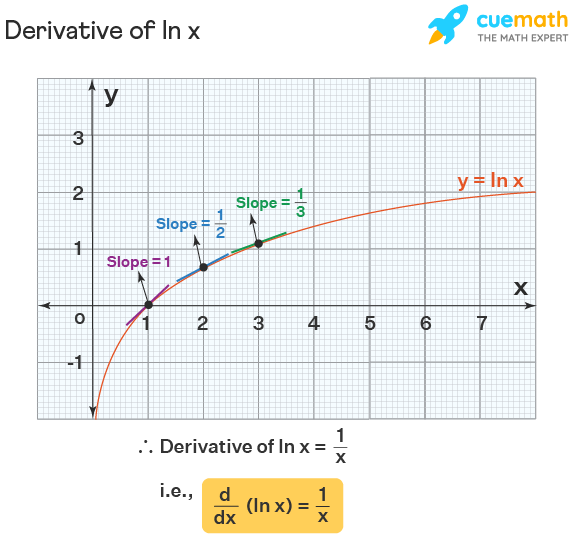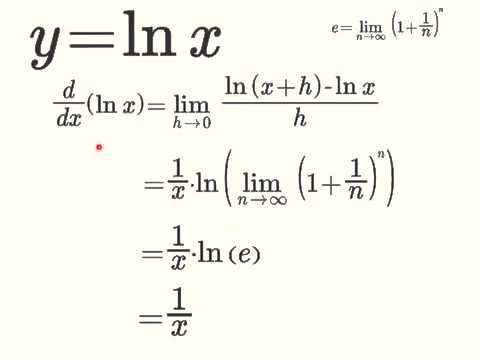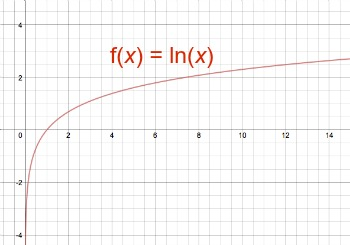Thursday, 6 Oct 2022

# The derivative of ln x- All you need to know

In the context of science and mathematics, derivatives is a very well known topic. In fact, students worry about this topic in general. However, it is not hard to learn. And, once you have mastered the ways of solving these problems, it is no longer an issue. In this article, we shall discuss the concept of derivatives in general. However, the main area of focus shall be finding the derivative of ln x.The changing rate of change of a function with regard to an explanatory variable is defined as a derivative. People usually use the derivative when there is a variable quantity and the rate of change is not fixed. The derivative is a tool for determining the reactivity of one variable (the dependent variable) to another one (independent variable). In this post, we’ll go over what derivatives are, how they’re stated in math, and how we can find the derivative of ln x.

## The derivative of ln x

Of course, ln x is a natural logarithmic function, as we know. It signifies that “ln” is for “logarithm with base e.” ln e = log e, for example. However, there are two ways to find the derivative of ln x.

Making use of the first principle of derivatives (definition of derivative)

And, through the use of implicit differentiation

Let’s look at what the derivative of ln x is. And, how to prove it using two methods and a few instances.

Firstly, we shall focus on the graph of the function of ln x. Then,we shall move on to find its derivative as well.

1/x is the derivative of ln x, that is,

For example, d/dx (ln x) = 1/x.

In other words, the natural logarithm of x has a derivative of 1/x. But, that is just a statement. Now, we have to prove it.

So, let us first prove that the derivative of ln x is 1/x in a rough way, using its graph.

Firstly, we can graph the function f(x) = ln x. As we all know, the slope of the tangent drawn to the graph of the function at that point is what we call the derivative of a function at a point. The slope of the tangent sketched is readily visible.

At x = 1, it’s one, at x = 2, it’s half, at x = 3, it’s a third, and so on.

This diagram shows the graph of ln x. Refer to this figure in order to understand the concept of the derivative of ln x better.Thus, it is clear that d/dx (ln x) = 1/x.

Also, we can write it as:

(ln x)’ = 1/x.

## Find the derivative of ln x

Now, we shall prove the formula that we have written in the earlier section. In fact, we shall use two different methods.

First, we begin by using the first principle method to find the derivative of ln x.

In fact, the first principle of derivatives is the real definition of derivatives.

Firstly, we consider the function itself:

f(x) = ln x.

Next, we need to define the first principle of derivatives.

In mathematics, f’(x) shows the derivative of f(x).

And, it is given by the limit:

f'(x) = limₕ→₀ [f(x + h) – f(x)] / h.

Now, in this case f(x) = ln x.

Thus, f(x+h) = ln (x+h).

The next step entails putting these values of f(x) and f(x+h) in the first principle formula.

Thus,

f'(x) = limₕ→₀ [ln (x + h) – ln x] / h.

Of course, by the logarithm subtraction property,

We have ln p – ln q = ln (p/q).

Using that, we can write

f'(x) = limₕ→₀ [ln [(x + h) / x] ] / h.

So, we substitute (h/x) = s.

Using this value boils it down to lims→₀ 1/(xs) ln (1 + s).

Using logarithm property, you can once again rewrite this to:

lims→₀ [ln (1+s)] ^ (1/xs).

Applying exponents formula,

f'(x) = limₜ→₀ ln [(1 + s)1/s]1/x

Now, we apply (ln a)^n = n ln a,

f'(x) = limₜ→₀ (1/x) ln [(1 + s)1/s]

Of course, we can write (1/x) outside of the limit.

Thus, f'(x) = (1/x) lims→₀ ln [(1 + s)1/s] = (1/x) ln lims→₀ [(1 + s)1/s]

According to the limits formula,  lims→₀ [(1 + s)1/s] = e. Therefore,

f'(x) = (1/x) ln e = (1/x) (1) = 1/x. (since, ln e = 1).## Prove the derivative of ln x

In the earlier section, we proved the derivative of ln x using the first principle method. Now, we shall use the implicit function method to prove the same.

This method is quite simple and short. But, we need a brief idea of the chain rule of derivatives for this one.

Firstly, let us assume z = ln x.

Thus, we can replace it by its exponent form.

For example, e^z = x.

Next, we have to find the derivatives on both the sides of the function.

Thus,

d/dx (ez) = d/dx (x).

Now, we apply the concept of chain rule to this.

Thus, e^z dz/dx = 1

Further, dz/dx = 1/e^z.

But, we know that ez = x.

So, we have proved that dz/dx = 1/x.

Finally, we have proved the derivative of ln x using the concept of implicit differentiation.

## The derivative of ln x is an odd function

Often, students are asked this question on tests:

Is the derivative of ln x an odd function?

And, they need to answer with either a true or false.

More often than not, they get it wrong. So, we shall discuss the way to check for it in order to make it easier for them to solve it.

Firstly, we need to say that the statement is false. That is, the derivative of ln x is not an odd function.

ln x is actually an odd function.

This means that ln (-x) = -1/x.

Even function, geometrically, denotes reflection down the y axis. Thus, any direction will reflect, implying that the derivative on the right is similar to the derivative on the left hand side, but the orientation will change. It signifies that the value will exactly be the same, but the sign is totally different.

Odd functions are rotationally symmetric. For instance, if you flip an arrow, or change its direction by 180 degrees, the slope remains the same. Likewise, the derivative of an odd function is always even.

Thus, we can safely conclude that the derivative of ln x is not odd. In fact, it is an even function. As ln x itself is an odd function. And, according to the premise made, the derivative of an odd function is always even.

## The nth derivative of ln x

Finding the nth derivative of ln x is as easy as finding its first derivative.

First, let us assume that z = ln(x)

Thus, we have z’ = z

The first derivative of ln x = 1/x

Or, x^(-1).

Thus, z” = (-1)*x^(-2) = (-1)^(2–1) * (2–1)! * x^-2

We can further write it as:

z”’ = z= (-1)*(-2)*x^-3 = (-1)^(3–1) * (3–1)! * x^-3

Now, we have to repeat this same process for n steps.

Thus, we can say that the nth derivative is:

Z [n] = d n z/ dx n = ( -1) ^ (n – 1) * (n – 1)!  * x^ -n.

Hence, we have obtained the nth derivative of ln x as well.

## The derivative of ln x^2

Finding the derivative of ln x^2 is very easy to find. However, we need to know the basics of the chain rule of differentiation in order to solve this one.

In fact, this is the process that we need to follow. We shall do it for you:

Let t = ln x^2.

So, dt/dx = 1 / (x^2) * d/dx (x^2).

Of course, we know that d/dx (x^2) = 2x.

Thus, we can further write it as,

dt/dx = 1 / (x^2) * 2x.

The x term cancels from the numerator and the denominator. However, one x term remains in the denominator. Thus, the final result becomes:

dt/dx = 2/x.

Thus, finding the result was pretty easy. We just need to know the basic elements of using the chain rule of derivatives. And, we are good to go.

## The derivative of ln (ln (x))

Similar to the previous derivative, we shall use the chain rule for this one as well.

In fact, it is that simple. The method is to keep finding the derivatives of any differentiable function at any point. And, finally we just calculate its product.

We represent this in the form :

d/dx f[g(x)] = f ’ [g(x)] g ‘ (x).

We call the f function in this case the external ln. Likewise, we call the internal g function as the internal ln.

We have to follow the same steps.

Firstly, let y = ln ( ln (x)).

Thus, dy / dx = (1 / ln x) * d/dx (ln x).

Earlier, we had already calculated the derivative of ln x. Thus, we will simply substitute:

d/dx (ln x) = 1/x.

So, finally it looks like:

dy / dx = (1 / ln x) * (1 / x).

The final result is dy/dx = 1 / (x ln x).

As you can see, finding the derivatives of log functions is actually pretty easy.

## The derivative of ln (x/y)

We use the same concept of using the chain rule to find the derivative of ln (x/y).

Thus, d/dx ( ln(x/y)) = { 1 / (x/y) } * d/dx (x/y).

We know what we will find when we differentiate (x/y).

However, we shall mention it for you:

d/dx (x/y) = 1/y.

Thus, the result now becomes:

d/dx ( ln(x/y)) = { 1 / (x/y) } * 1/y

Or, y/x * 1/y

Or, 1/x.

Thus, the answer simply becomes 1/x.

## The derivative of ln (cos x) is

Now, it is time to find the derivatives for the logarithms of trigonometric functions.

So, we shall find the derivative of ln (cos (x)).

However, it is not very much different from the earlier cases.

For example, we have to apply the same chain rule method to solve this derivative as well.

Firstly, we assume that y = ln (cos x).

Thus, dy/dx = 1 / cos x * d/dx (cos x).

For this, we have to know the derivative results of some basic trigonometric functions like cos x, sin x and tan x.

For example, d/dx (cos x) = -sin x.

Thus, dy/dx = 1 / cos x * (- sin x).

The final result looks like – sin x/ cos x.

We can also simply write this as – tan x ( since, {sin x / cos x} = tan x).

## Find the derivative of ln(x^2 + 1)

Finding this result is very easy. In order to find the derivative of this function, use the chain rule once again.

Let y = ln(x^2 + 1).

Thus, dy/dx = {1/(x^2 + 1)} * d/dx( x^2 + 1)

Now, according to rules of derivatives, d/dx ( f(x) + g(x) )= d/dx (f(x)) + d/dx (g(x)).

Thus, we can break the equation further as;

dy/dx = {1/(x^2 + 1)} * [d/dx( x^2) + d/dx ( 1)]

Or, {1/(x^2 + 1)} * 2x ( since, the derivative of any constant is 0).

So, the final result is {2x / (x^2 + 1)}.

Hence, you can see that if you can grasp the concept of chain rule, you can very easily solve the derivatives of any of the logarithmic functions.

## What is the derivative of ln x^3

You have to follow a similar method as finding the derivative of ln x^2.

Basically, set y = ln x^3.

So, dy/dx = 1 / (x^3) * d/dx (x^3).

Of course, we know that d/dx (x^3) = 3x.

Thus, we can further write it as,

dy/dx = 1 / (x^3) * 3x.

The x term cancels from the numerator and the denominator. However, one x term remains in the denominator. Thus, the final result becomes:

dt/dx = 3/x.

As you can see, the structure is totally the same as getting the derivative of ln x^2.

Thus, we know that the answer is correct.

## what is the derivative of ln(xy)

This is somewhat different from finding any other derivative.

Let s = ln (xy)

Or, e^s = xy

So, we can make (xy) explicit:

y(x) = e^x / x

And, dy/dx = (xe^x – e^x) / x^2

Or, e^x ((x-1) / x^2).

This is the final result for the derivative of ln (xy).

## The derivative of ln x FAQs

### 1.   How do you derive the derivative of ln x?

Ans: There are various ways to find the derivative of ln x. Firstly, you can draw a graph to find the result as we have done before. Then, you can apply the first principle of derivatives to obtain the same result. Also, we have used the implicit differentiation method to obtain the exact same result. This is basically the chain rule method. So, you can easily do that. Since the domain of ln x is x > 0, thus, d/dx (ln |x|) = 1/x.

No matter what method you use, the derivative of ln x will always be 1/x.

### 2.   What happens when you take the derivative of ln?

Ans: When you take the derivative of ln, you have to act according to the basic rules of differentiation. For any logarithmic function, the part after ln, goes to the denominator in its derivative form. Further, if the denominator is still a function of x, we have to keep on applying chain rule until that is the case.

However, the derivative of ln x is 1/x.

### 3.   What does ln x integrate to?

Ans: Much like derivatives the concept of integration for logarithmic functions is also quite common. The formula for the same is pretty simple.

We write the formula for the integral of ln x as, ∫ln x dx = xlnx – x + C, where C is the constant of integration.

### 4.   What is the derivative of x ln X?

Ans: x ln x’s derivative is equal to ln x + 1. We can use the product rule to compute this derivative. In fact, we do so by considering x as the first function and ln x as the second function. As x ln x is the product of x and ln x and the equation for the derivatives of x and ln x.

### 5.   How do you draw ln x on a graph?

Ans: We use the symbol ln instead of log e (x) to represent the natural logarithm (x). To graph f(x) = ln, we’ll use the following features of the graph of f(x) = log a (x) (x). The graph’s x-intercept, or the point where the graph cuts the x-axis, is (1, 0). The graph’s vertical asymptote is represented by the y-axis.

However, the graph looks like this:### 6.   How do you find the log of a graph?

Ans: Determine the coordinates of two points on the line. Calculate the difference between these two points’ y-coordinates (rise). Then, calculate the x-coordinate difference between these two places (run). Finally, divide the y-coordinate difference by the x-coordinate difference (rise/run or slope).

### 7.   How to make a ln graph in Excel?

Ans: Excel comes in very handy while arranging data. So, you might want to draw a graph in excel as well. If the graph is a ln one, try these steps:

• Open your xy scatter graph. Then, click on the format axis after clicking on each axis.
• Under the format axis bar, click on the axis options tab and finally click on the logarithmic scale.

However, follow these steps for excel version 2007 and 2010.

For other version users:

• You have to double click each axis on your xy scatter graph.
• Go to the format axis bar. Then, select the scale tab and finally choose the logarithmic scale from there.

And, you can create ln graphs on Excel that simply.